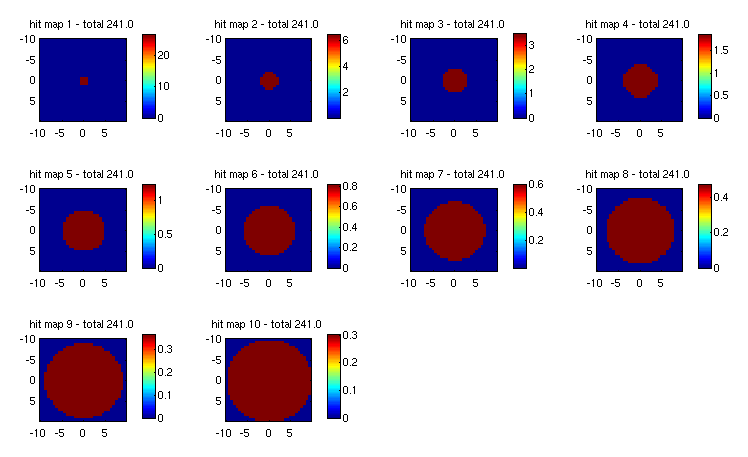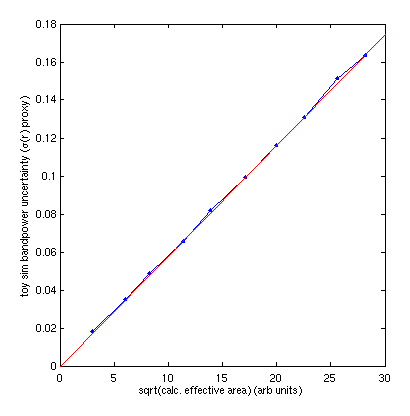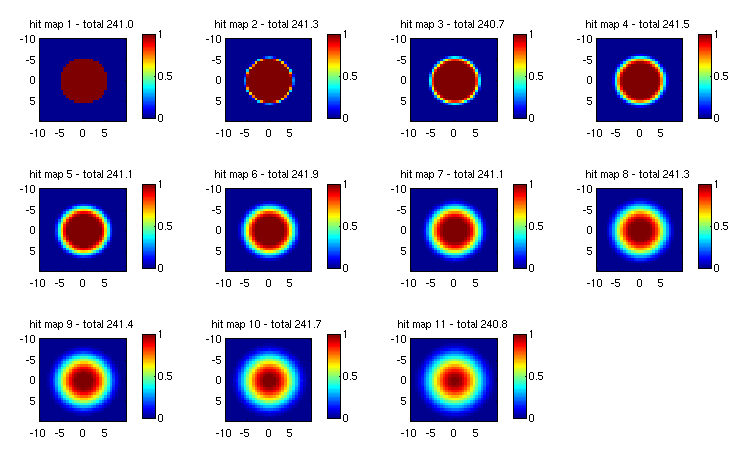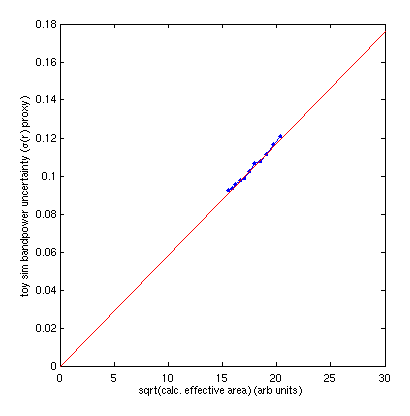## Intro

People often talk about effective $$f_\mathrm{sky}$$ for a given observation hit pattern. I think they may sometimes define it differently. Recently we have been calculating it from the fractional fluctuation of simulated bandpowers. For the three CMB-S4 hit patterns we have been considering I did this in Sims_with_nominal_Chile_and_Pole_masks_II. - see the numbers in the panel titles in the second plot. The number one gets depends on whether signal or noise bandpowers are considered with noise producing larger numbers since it "resists apodization".

The SO projections paper states that $$f_\mathrm{sky}^\mathrm{eff}=\langle N_\mathrm{hits} \rangle^2 / \langle N_\mathrm{hits}^2 \rangle$$. Here I calculate this number for the three masks in question and then do some simple numerical experiments to verify that bandpower uncertainty scales linearly with the square-root of this quantity (and hence that $$\sigma(r)$$ can be expected to do so in the noise limited regime).

## Calculated $$f_\mathrm{sky}^\mathrm{eff}$$ for Circular/Chile/Pole masks

The hit maps are shown in Sims_with_nominal_Chile_and_Pole_masks.

The numbers can be compared as:

                       Circular Chile  Pole
frac fluc noise sims  0.041    0.197  0.030
expression above      0.036    0.185  0.027
(f non zero            0.054    0.349  0.049)


There is Monte Carlo noise in the first set of numbers. I think we can conclude there is no evidence for difference between the first and second set. Some difference might be excused due to the E/B purification which occurs in the sims. However, since the ratios are pretty flat with $$\ell$$ (and the numbers above are an average dominated by higher $$\ell$$) I doubt that has much effect.

## Toy Sims

This follows on from 20181105_toy_sims.

I first take a set of 10 simple binary circular hit maps with equal sums:

Fig 1:I generate noise realizations for these, apodize by the hit map, take the variance, and then take the standard deviation of that to get a proxy for noise bandpower uncertainty - and in the noise limited regime $$\sigma(r)$$. I then calculate $$f_\mathrm{sky}^\mathrm{eff}$$ from the expression given above, but since the patterns are uniform discs this is the same as just taking the non-zero area. Plotting the square-root of the area versus the $$\sigma(r)$$ proxy we recover the expected result that for a fixed amount of experimental effort sigma(r) increases with the square-root of the observed sky area:

Fig 2:I now switch to considering a set of hit maps with varying taper:

Fig 3:I repeat the toy sim and again calculate $$f_\mathrm{sky}^\mathrm{eff}$$ from the expression given above and plot the square-root of the area versus the $$\sigma(r)$$ proxy. We see below that the relationship is again linear. It is interesting how small the range of $$f_\mathrm{sky}^\mathrm{eff}$$ is for such different looking masks.

Fig 4:here